Note: This is an archvied version of our old webpage. Some links might be broken. The current one can be found here.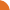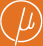Chair for Foundations of Software Reliability and Theoretical Computer Science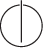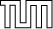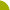Publications - Minimisation of Multiplicity Tree AutomataReference:

Stefan Kiefer, Ines Marusic, and James Worrell. Minimisation of multiplicity tree automata. Logical Methods in Computer Science (LMCS), 13(1), 2017.

Abstract:

We consider the problem of minimising the number of states in a multiplicity tree automaton over the field of rational numbers. We give a minimisation algorithm that runs in polynomial time assuming unit-cost arithmetic. We also show that a polynomial bound in the standard Turing model would require a breakthrough in the complexity of polynomial identity testing by proving that the latter problem is logspace equivalent to the decision version of minimisation. The developed techniques also improve the state of the art in multiplicity word automata: we give an NC algorithm for minimising multiplicity word automata. Finally, we consider the minimal consistency problem: does there exist an automaton with n states that is consistent with a given finite sample of weight-labelled words or trees? We show that this decision problem is complete for the existential theory of the rationals, both for words and for trees of a fixed alphabet rank.

Suggested BibTeX entry:

@article{17KMW-LMCS,
author = {Stefan Kiefer and Ines Marusic and James Worrell},
journal = {Logical Methods in Computer Science (LMCS)},
number = {1},
title = {Minimisation of Multiplicity Tree Automata},
volume = {13},
year = {2017}
}See doi.org ...Conference version